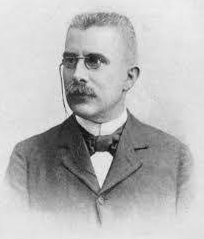Study the Shift in Equilibrium between [Co(H₂O)₆] ²⁺ and Cl⁻ ions

# Our Objective

To understand the process of shift in equilibrium between [Co (H2O)6] 2+ and chloride ions by changing the concentration of either of the ions.

# The Theory

## What is Chemical equilibrium?

Chemical equilibrium refers to the state of a system in which the concentration of the reactant and the concentration of the products do not change with time and the system does not display any further change in properties.

The equilibrium reaction between [Co(H2O)6]2+ and chloride ions through the change in the concentration of the ions. Following displacement takes place in the chemical reaction between [Co(H2O)6]2+ and chloride.Consider as a general reaction for the equilibrium constant

A + B → C + D is given as:The expression for the equilibrium constant for the reaction between [Co(H2O)6]2+ and chloride ions is given as:Hence, the reaction takes place in an aqueous medium where the concentration of H2O remains constant.

At equilibrium either [Co(H2O)6] 2+ ion or Cl – ions concentration is increased, and this would result in an increase in [CoCl4] 2– ion concentration thus, maintaining the value of K as constant. There is a shift in the equilibrium in the forward direction along with the change in colour.

## Law of Equilibrium?

The Law of Chemical Equilibrium is defined as the ratio of the product of the concentration of the products to the product of the concentration of the reactants, with each concentration term raised to the power by its coefficient in an overall balanced chemical equation; it is a constant quantity at a given temperature, and it is called equilibrium constant.

## What is Le Chatelier's principle?Le Chatelier, a French chemist in 1884, proposed that the effect of change in temperature, pressure and concentration on a system in equilibrium can be studied. Le Chatelier’s principle states that the new mixture is also called equilibrium when we add chemicals together than the number of molecules in each chemical. In a chemical reaction, there are always different numbers of atoms and molecules. There are some rules about how these numbers should change when you mix chemicals. For example, if two gasses combine, the total gas amount must stay the same. That means the number of molecules in the mixture stays the same. This is known as a chemical equilibrium.

Temperature, pressure, and concentration all affect the state of equilibrium in a system. Change to any of them, or change how much of each there is, and you’ll see equilibrium being disrupted. Equilibrium is restored when everything goes back to normal.

The principle of Le Chatelier is an observation regarding the chemical equilibria of processes. It asserts that changes in a system’s temperature, pressure, volume, or concentration will cause predictable and opposing changes to attain a new equilibrium state. In practice, Le Chatelier’s approach may be utilised to understand reaction circumstances that encourage enhanced product production.

Let us consider the example;

## 2SO2 (g) + O2 (g) ⇌ 2SO3 (g); ΔH = −189kJ

Effect of Temperature: Because the enthalpy of SO3 production is negative, the above equilibrium reaction is exothermic. When the temperature of the reaction is raised, the reaction will proceed backwards in the direction of heat absorption. When the temperature is lowered, the equilibrium shifts forward, causing heat to be released. Low temperature promotes this reaction due to its exothermic nature. For the Contact method, an ideal temperature of 673-723 K is used to produce SO3

Pressure's effect:  The number of moles decreases while the reaction is performed. According to Le Chatelier, if the pressure rises, the equilibrium moves in the direction where the pressure falls. As a result, the number of moles decreases in the forward direction.  So, as pressure rises, SO3 production is increased.

Effect of Concentration: According to Le Chatelier's principle, as the concentrations of the reactants SO2 and O2 rise, the reaction equilibrium shifts in the direction of reactant consumption, i.e. forward. As a result, increased SO2 and O2 concentrations result in increased SO3

# Learning Outcomes

• Students understand the term ‘chemical equilibrium’ through the experiment.
• Students understand the effect of change in concentration on the equilibrium of a reaction.
• Students understand the shift in equilibrium position with respect to the concentration change.
• Students are better able to perform the experiment in the real lab after experiencing it in the virtual lab.